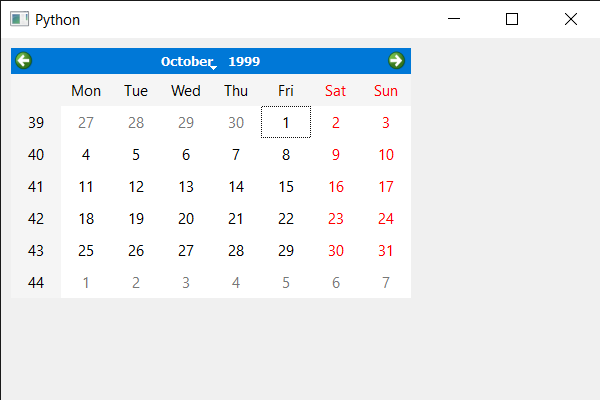Open In App

# PyQt5 QCalendarWidget – Setting Current Page

In this article we will see how we can change/set the current page of the QCalendarWidget. Page in the QCalendarWidget is the selected month of given year, changing page will also change the month as well as year.

In order to do this we will use setCurrentPage method with the QCalendarWidget object.
Syntax : calendar.setCurrentPage(year, month)
Argument : It takes two integer as argument i.e year and month
Return : It return None

Below is the implementation

## Python3

 `# importing libraries``from` `PyQt5.QtWidgets ``import` `*``from` `PyQt5 ``import` `QtCore, QtGui``from` `PyQt5.QtGui ``import` `*``from` `PyQt5.QtCore ``import` `*``import` `sys`  `class` `Window(QMainWindow):` `    ``def` `__init__(``self``):``        ``super``().__init__()` `        ``# setting title``        ``self``.setWindowTitle(``"Python "``)` `        ``# setting geometry``        ``self``.setGeometry(``100``, ``100``, ``600``, ``400``)` `        ``# calling method``        ``self``.UiComponents()` `        ``# showing all the widgets``        ``self``.show()` `    ``# method for components``    ``def` `UiComponents(``self``):` `        ``# creating a QCalendarWidget object``        ``calendar ``=` `QCalendarWidget(``self``)` `        ``# setting geometry to the calendar``        ``calendar.setGeometry(``10``, ``10``, ``400``, ``250``)` `        ``# year and month``        ``year ``=` `1999``        ``month ``=` `10` `        ``# setting current page``        ``calendar.setCurrentPage(year, month)`    `# create pyqt5 app``App ``=` `QApplication(sys.argv)` `# create the instance of our Window``window ``=` `Window()` `# start the app``sys.exit(App.``exec``())`

Output :